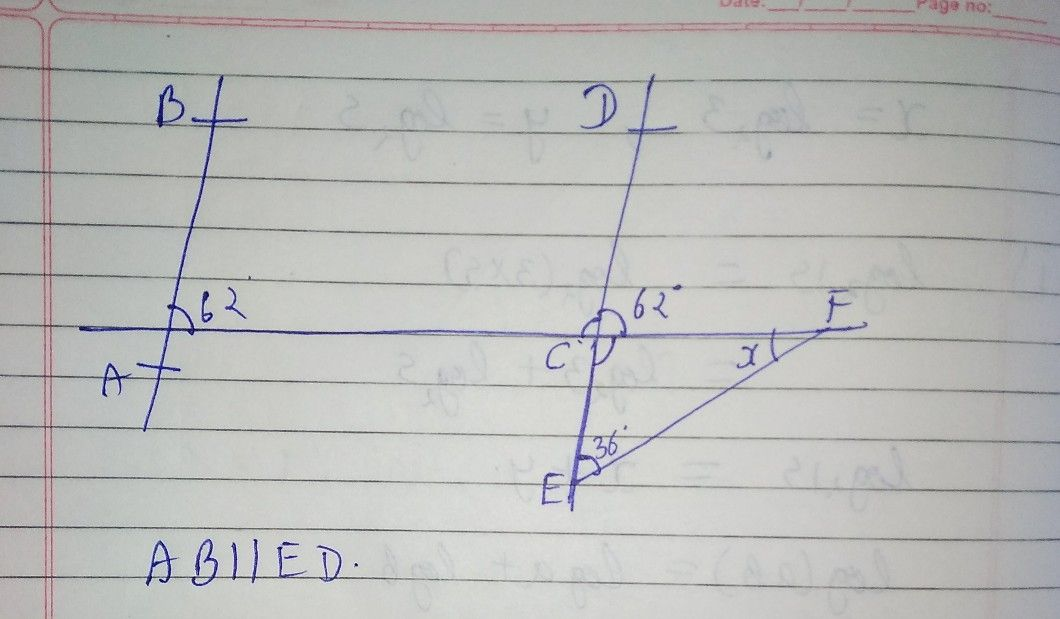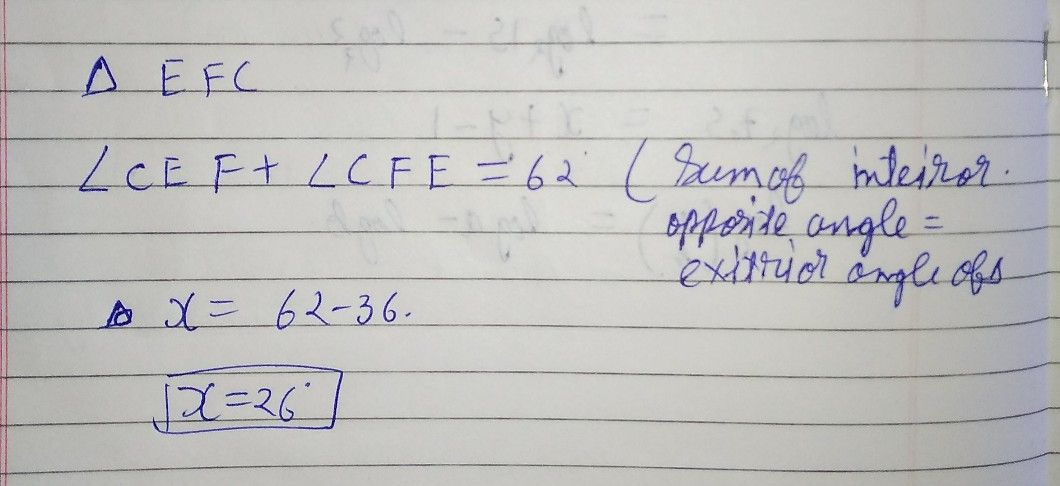Symbol
ProblemQuestion $32$ Not $y$ yet answered Marked out of $100$ In figure, $AB|1$ $ED$ the value of $x$ $1s:$ $B$ $1$ $0y$ $62^{°}$ $62^{°}$ $E$ $\bar{A}$ $C$ $x$ $63$ $E$
7th-9th grade
Geometry
SolutionQanda teacher - MeenaIf you have any doubt in given problem feel free to ask:) Dear, Please evaluate the answer and give gift coins(it does not reduce your coin balance.) for giving gift coins Click on evaluate. Then press the + icon 3 times(3000)Student
thnx mam